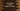# C++ program to count the total number of digits in a string# Introduction :

In this tutorial, we will learn how to count the total number of digits in a string in C++. The program will take a string as input from the user and prints out the result.

The user can enter any type of string. It can include numbers, special characters, space, tabs or any other character. For example, if the string is “abc1234”, it will print 4 as the output.

With this program, you will learn how to use functions in C++, how to check if a character is a number or not, how to use a for loop, how to use if-else condition, and how to print a message to the user in C++.

Let’s take a look at the program :

## C++ program :

``````#include <iostream>
#include <ctype.h>
using namespace std;

//1
bool isNumber(char c)
{
if (isdigit(c))
return true;
return false;
}

//2
int main()
{
//3
string str;
int count = 0;

//4
cout << "Enter a string : ";
getline(cin, str);

//5
for (int i = 0; i < str.size(); i++)
{
//6
if (isNumber(str[i]))
{
//7
count++;
}
}

//8
cout << "Total number of digits : " << count << endl;

return 0;
}``````

## Explanation :

The commented numbers in the above program denote the step numbers below :

1. isNumber function is used to check if a character is number or not. It takes one character as the argument and returns one boolean value. true if it is a number and false otherwise.
2. main() is the starting point of the program. This method is executed first.
3. Inside the main method, we are defining one string str and one integer count variable. The str variable is for holding the user input string and count is for holding the total count of numbers in the string.
4. Ask the user to enter a string. cout is used to print a message to the user.
5. Run one for loop to iterate over the string one by one character. It will run from i = 0 to i = length of the string - 1. On each iteration, the value of i will increase by 1.For example, for the string “hello”, it will run from i = 0 to i = 4.We can access the characters of a string with its index. The index starts from 0 and ends on length - 1.
6. Inside the loop, on each iteration, check if the current character is a number or not. We are using str[i] to access the current character. For the first iteration, i is 0, so it will verify the first character, for the second iteration, i is 1 and it will verify the second character and so on.
7. If the current character is number or the return value of isNumber function is true, increment the value of the count variable by 1. Its initial value was 0. So, if we find the first number, it will be 1, for the second number it will be 2 and so on.
8. At the end of the program, count will hold the total count of numbers in the string. Print this value to the user. Notice how we are concatenating the message text with the integer count in a single cout line.

### Sample Output :

``````Enter a string : hello 12world
Total number of digits : 2

Enter a string : 12345678910
Total number of digits : 11``````

### Conclusion :

In this tutorial, we have learned how to count the total numbers in a string. You can use the same approach to count the total characters in a string. Try to implement this and don’t hesitate to drop one comment below if you have any queries.